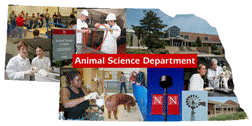## Animal Science Department## Faculty Papers and Publications in Animal Science

#### Date of this Version

January 1993

Published in J. Anim. Sci. 1993. 712336-844.

#### Abstract

Transformation of multiple-trait records that undergo sequential selection can be used with derivative-free algorithms to maximize the restricted likelihood in estimation of covariance matrices as with derivative methods. Data transformation with appropriate parts of the Choleski decomposition of the current estimate of the residual covariance matrix results in mixed-model equations that are easily modified from round to round for calculation of the logarithm of the likelihood. The residual sum of squares is the same for transformed and untransformed analyses. Most importantly, the logarithm of the determinant of the untransformed coefficient matrix is an easily determined function of the Choleski decomposition of the residual covariance matrix and the determinant of the transformed coefficient matrix. Thus, the logarithm of the likelihood for any combination of covariance matrices can be determined from the transformed equations. Advantages of transformation are 1) the multiple-trait mixed-model equations are easy to set up, 2) the least squares part of the equations does not change from round to round, 3) right-hand sides change from round to round by constant multipliers, and 4) less memory is required. An example showed only a slight advantage of the transformation compared with no transformation in terms of solution time for each round (1 to 5%).

COinS